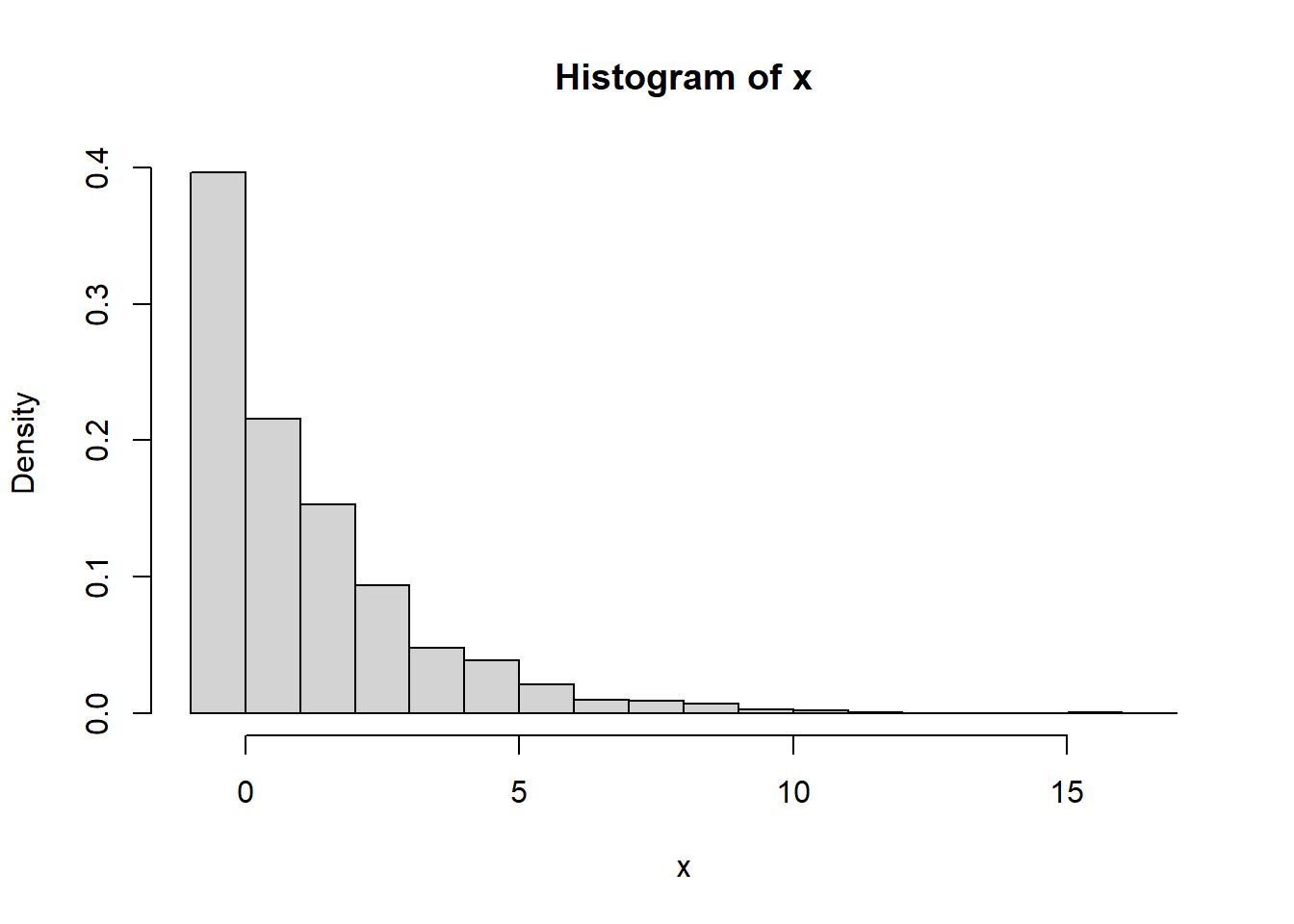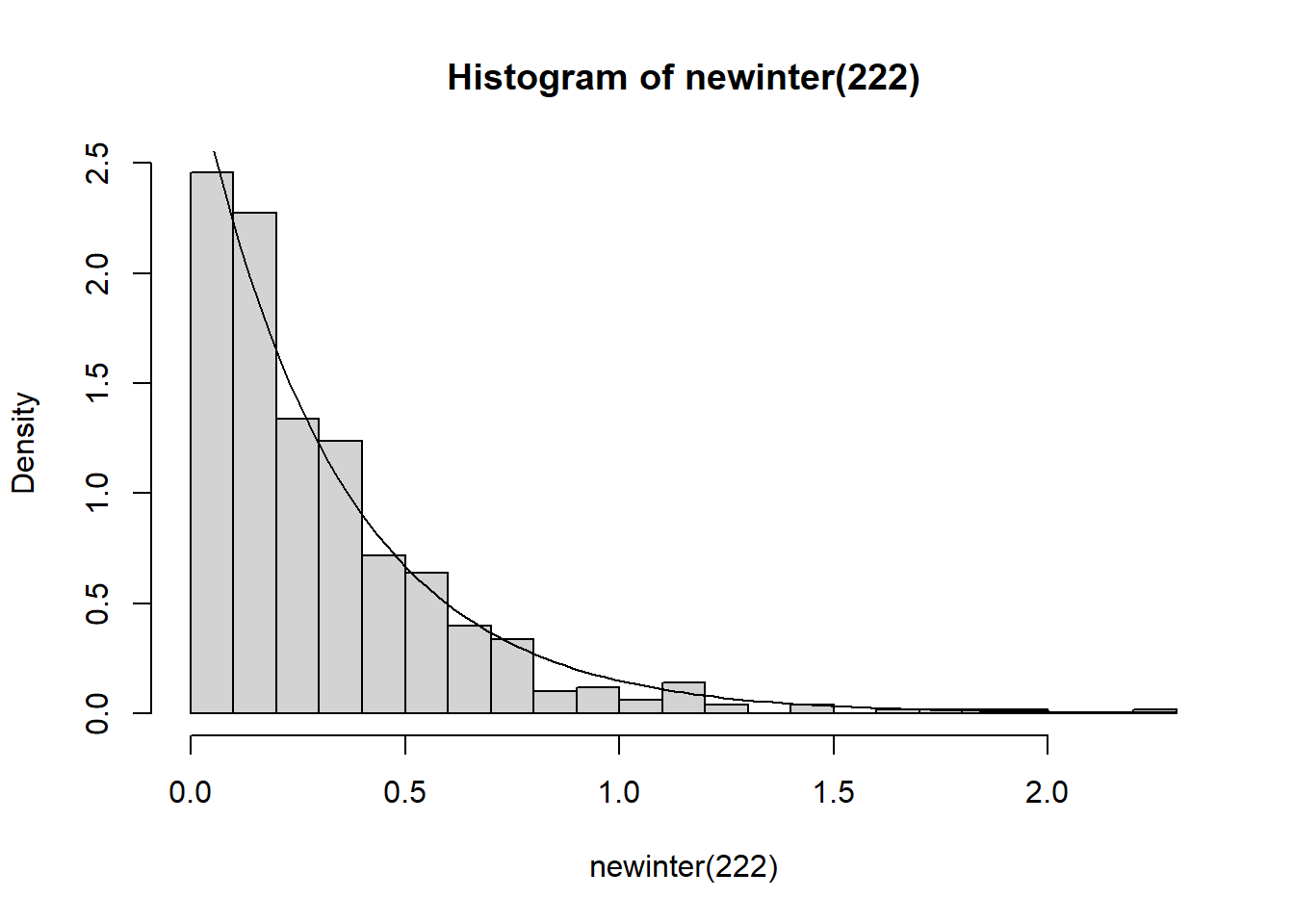Exercise 1 Use the alternating renewal theorem to show that under mild conditions, we have that the probability that the number of arrrival in the interval $$[0,t]$$ is even goes to $$1/2$$ as $$t \to \infty$$. Why do we need mild conditions? Why do we need $$t \to \infty$$.

Solution.

• We can not expect the limit to converge to $$1/2$$ if we have a lattice type distribution. In particular, imagine the case where the inter-arrival times are deterministic and occur at integer times; in the case we know exactly when it will be even or odd. In general, even we also know near the beginning there are no arrivals, so it will more likely be even; thus we need to consider large $$t$$.

• If we envision the inter-arrival times as an alternating process, where the working time has the same distribution as the service time, we are are interested when the machine is working, and this limit goes to $$1/2$$ by the alternating renewal theorem

Exercise 2 Consider a $$M(3)/M(5)/1$$ queue. Let $$t=323$$, so the queue has had a chance to settle in.

• By running simulations, plot the probability mass function for the random number of customers in the system. Do you recognize it? Any guesses?

• Start keeping track of the departures and assume they proceed into a new shop. By simulations, produce a histogram of the inter-arrivals times of the items entering the new shop. You might be surprised.

Solution. First, we produce code that checks the number of items in the system at time $$t$$. We make modfication to our previous code on queues.
num <-function(t){
inter = rexp(1,3)
arr = inter

while(t > arr[length(arr)]){
inter <-c(inter, rexp(1,3))
arr <- cumsum(inter)
}

L = length(inter)
service = rexp(L, 5)

output <- arr + service
for (i in 1:(L-1) ) {
if (arr[i+1] < output[i]){output <- c(output, output[i] + service[i+1])}
if (arr[i+1] > output[i]){output <- c(output, arr[i+1] + service[i+1])}
}

output <- output[-L]

n=sum(output >t)

n
}
num(323)
##  0
x = replicate(1000, num(323))
b = seq(-1,max(x)+1, by=1)
hist(x, prob=TRUE, breaks=b)Checking your favorite discrete distributions, you might guess that this is geometric (the version that allows $$0$$), with parameter $$(1- 3/5)$$. A preliminary test shows:

p= 1-3/5

mean(x) - (  (1-p)/p )
##  0.102
var(x) -  (  (1-p)/p^2 )
##  0.4357818
testt= c(sum(x==0)/1000 - dgeom(0,p),sum(x==1)/1000 - dgeom(1,p)
,sum(x==2)/1000 - dgeom(2,p),sum(x==3)/1000 - dgeom(3,p),
sum(x==4)/1000 - dgeom(4,p))

testt
##  -0.00400 -0.02400  0.00900  0.00760 -0.00384

We modify our code to give inter-arrival times from the departure times. We will take $$500$$ arrivals after the arrival just arriving after after $$t$$, and consider the interideparture of these arrivals. It may come as a surprise, that new inter-arrivals are exponenital with rate $$3$$, which is what we started with.

newinter <- function(t){
inter = rexp(1,3)
arr = inter

while(t > arr[length(arr)]){
inter <-c(inter, rexp(1,3))
arr <- cumsum(inter)
}

L = length(inter)

inter <- c(inter, rexp(500, 3) )
arr = cumsum(inter)

service = rexp((500+L), 5)

output <- arr + service
for (i in 1:(L+499) ) {
if (arr[i+1] < output[i]){output <- c(output, output[i] + service[i+1])}
if (arr[i+1] > output[i]){output <- c(output, arr[i+1] + service[i+1])}
}

times =  output[L] - output[L-1]
for (i in 0:499){
times <- c(times, output[L+1+i] - output[L+i] )
}
times
}
hist(newinter(222), prob=TRUE, breaks=30)
curve(dexp(x,3), add=TRUE)Exercise 3 (Little’s law) There are many simple exercises on can do with Little’s law. See here